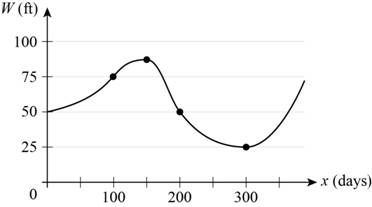# The average rate of change of water between x = 100 and x = 200 from the given graph.### Precalculus: Mathematics for Calcu...

6th Edition
Stewart + 5 others
Publisher: Cengage Learning
ISBN: 9780840068071### Precalculus: Mathematics for Calcu...

6th Edition
Stewart + 5 others
Publisher: Cengage Learning
ISBN: 9780840068071

#### Solutions

Chapter 2.4, Problem 23E
To determine

## To find: The average rate of change of water between x=100 and x=200 from the given graph.

Expert Solution

The average rate of change of water between x=100 and x=200 from the given graph is 0.25ft/day .

### Explanation of Solution

Given:

The given graphs is,Figure (1)

Formula used:

Average rate of change of function y=f(x) between x=a and x=b .

Averagerateofchange=ChangeinyChangeinx=f(b)f(a)ba (1)

Calculation:

The given graph shows the depth of the water in a reservoir over a one-year period as a function of number of days x.

From the given graph, for x=100 , W(ft) is 75 and for x=200 , W(ft) is 50.

Substitute 50 for f(b) , 75 for f(a) , 100 for a and 200 for b in equation (1).

Averagerateofchange=5075200100=25100=14=0.25ft/day

Therefore, the average rate of change of water between x=100 and x=200 from the given graph is 0.25ft/day .

### Have a homework question?

Subscribe to bartleby learn! Ask subject matter experts 30 homework questions each month. Plus, you’ll have access to millions of step-by-step textbook answers!